# Unbiased test

(diff) ← Older revision | Latest revision (diff) | Newer revision → (diff)
A statistical test of size (level),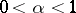, for testing a compound hypothesis: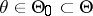against a compound alternative: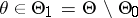, whose power function(cf. Power function of a test) satisfies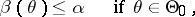In many problems in statistical hypotheses testing there are no uniformly most-powerful tests (cf. Uniformly most-powerful test). But if one restricts the class of tests, then there may be uniformly most-powerful tests in that class. If in the problem of testing the hypothesis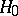against the alternative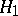there is a uniformly most-powerful test, then it is unbiased (cf. Unbiased test), since the power of such a test cannot be less than that of the so-called trivial test whose critical functionis constant and equal to the size, that is,, whereis the random variable whose realization is used to test the hypothesisagainst the alternative.
Example. The sign test is uniformly most-powerful unbiased in the problem of testing the hypothesisaccording to which the unknown true value of the parameterof the binomial distribution is equal toagainst the alternative: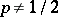.